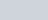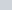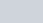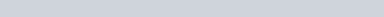# SSC CGL Reasoning Questions with Solutions

SSC CGL Reasoning questions with solutions in English. Question and answer with solution for online practice. Useful for preparation of govt jobs competitive exams.
Number of Questions : 25

## SSC CGL Reasoning Questions with Solutions

Reasoning SSC CGL Questions with solution (Hindi) Start here

Q.1: Select the set of classes, the relationship among which is best illustrated by the following Venn diagram.

(A) Stationery, Stapler, Erasers
(B) Doctors, Fathers, Sisters
(C) Bureaucrats, Men, Women
(D) Extroverts, Men, Handsome

Ans : (D) Extroverts, Men, Handsome

Q.2: Four number-pairs have been given, out of which three are alike in some manner and one is different. Select the number-pair that is different.
(A) (547, 258)
(B) (723, 144)
(C) (812, 121)
(D) (546, 225)

Ans : (A) (547, 258)
7+2+3 = 12, 122 = 144
8+1+2=11, 112 = 121
5+4+6 = 15, 152 = 225
5+4+7 = 16, 162 =256 (not 258)

Q.3: Select the option in which the numbers are related in the same way as are the numbers of the following set.
(12, 121, 169)
(A) (11, 144, 196)
(B) (14, 196, 225)
(C) (16, 161, 256)
(D) (17, 256, 324)

Ans : (D) (17, 256, 324)
(12, 121, 169) = 12, (12-1)2, (12+1)2
(17, 256, 324) = 17, (17-1)2, (17+1)2

Q.4: Select the letter-cluster from among the given options that can replace the question mark (?) in the following series.
MCN, NCO, OCP, PCQ, ?
(A) PQR
(B) QCR
(C) OCQ
(D) PCS

Ans : (B) QCR
+1, +0, +1

Q.5: Four words have been given, out of which three are alike in some manner and one is different. Select the word that is different.
(A) Diarrhoea
(B) Scurvy
(C) Goitre
(D) Anaemia

Ans : (A) Diarrhoea / डायरिया (दस्त)

Q.6: Two different positions of the same dice are shown, the faces of which are marked with the letters F, G, H, I, J and K. Select the letter that will be on the face opposite to the face having the letter ‘F’.

(A) I
(B) H
(C) J
(D) G

Ans : (D) G
Clockwise from J in both Dice$\frac JJ, \frac FG, \frac IH$

Q.7: Select the combination of letters that when sequentially placed in the blanks of the given series will complete the series.
JL_N_J_PND_NP_DJ_P_D
(A) M, D, N, P, N, J, O
(B) P, M, D, N, J, O, N
(C) P, D, M, J, N, O, N
(D) M, D, P, N, J, N, O

Ans : (C) P, D, M, J, N, O, N

Q.8: Select the number from among the given options that can replace the question mark (?) in the following series.
121, 169, 225, 289, ?
(A) 410
(B) 305
(C) 398
(D) 361

Ans : (D) 361
112, 132, 152, 172, 192

Q.9: Select the option figure that is embedded in the given figure (rotation is NOT allowed).

Ans : ( b. )

Q.10: Select the option in which the words share the same relationship as that shared by the given pair of words.
Pulmonologist : Lungs
(A) Endocrinologist : Glands
(B) Ophthalmologist : Ears
(C) Nephrologist : Nerves
(D) Bones : Orthopaedic

Ans : (A) Endocrinologist : Glands : एंडोक्रिनोलॉजिस्ट: ग्रंथियां

Q.11: Select the correct combination of mathematical signs that can sequentially replace the * signs and make the equation correct.
45 * 24 * 72 * 20 * 12 * 7
(A) =, x, +,$\div$ , –
(B) x,$\div$ , =, -, +
(C)$\div$ , x , +, -, =
(D) +,$\div$, -, =, x

Ans : (B) x,$\div$ , =, -, +

Q.12: Select the figure from among the given options that can replace the question mark (?) in the following series.

Ans : ( b. )

Q.13: Select the correct mirror image of the given combination when the mirror is placed at ‘PQ’ as shown.

Ans : ( b.)

Q.14: The monthly income of the tree cricketers Ankit, Sanjay and Roshan, from different sources, are in the ratio of 12 : 9 : 7, and their expenditures are in the ratio 15 : 9 : 8. If Ankit saves 25% of his income for future investments, what is the ratio of the savings of Ankit, Sanjay and Roshan ?
(A) 23 : 18 : 11
(B) 5 : 8 : 7
(C) 15 : 18 : 11
(D) 25 : 16 : 13

Ans : (C) 15 : 18 : 11
Ankit : Sanjay : Roshan :: Income 12x : 9x : 7x
Ankit : Sanjay : Roshan :: Expenditures 15y : 9y : 8y
Ankit : Sanjay : Roshan :: Savings 12x – 15y : 9x – 9y : 7x – 8y -(1)
Savings of Ankit = 12x – 15y = 25% of 12x = 3x
=> 9x = 15y,$\frac xy = \frac{15}{9}$
Put the value of x and y in (1)$(12\times15 - 15 \times9) : (9 \times 15 - 9 \times 9) : (7 \times 15 - 8 \times 9)$
45 : 54 : 33 => 15 : 18 : 11

Q.15: How many triangles are there in the given figure ?

(A) 7
(B) 8
(C) 9
(D) 10

Ans : (B) 8

Q.16: Select the option that is related to the fourth number in the same way as the first number is related to the second number and the fifth number is related to the sixth number.
11 : 81 : : ? : 121 : : 8 : 36
(A) 10
(B) 12
(C) 18
(D) 13

Ans : (D) 13
(11-2)2 = 81
(13-2)2=121

Q.17: Study the given pattern carefully and select the number that can replace the question mark (?) in it.

(A) 21
(B) 23
(C) 18
(D) 19

Ans : (A) 21
(34-12)x5 =110
(13-6)x5=35
(n-9)x5 =60 =>5n =60+45 = 105
=> n=105/5 =21

Q.18: Read the given statements and conclusions carefully. Assuming that the information given in the statements is true, even if it appears to be at variance with commonly known facts, decide which of the given conclusions logically follows from the statements.

Statements:
All lockets are chains.
100% medals are lockets.
Some rings are chains.
Conclusions:
I. All chains are medals.
II. All medals being rings is a possibility.
(A) Only conclusion II follows
(B) Neither conclusion I nor II follows
(C) Only conclusion I follows
(D) Both conclusions I and II follow

Ans : (A) Only conclusion II follows

Q.19: There are two couples in a family. Komali has two children. Madhurima is the wife of omprakash, who is the brother of Mani. Pinki is the daughter of kamali. Urmila is the sister of Sanju, who is the son of Omprakash. Tarun is the son of Mani, who is a male.
How is Madhurima is related to Komali ?
(A) Sister-in-low
(B) Mother
(C) Sister
(D) Aunt

Ans : (A) Sister-in-low

Q.20: Select the correct option that indicates the arrangement of the given words in the order in which they appear in an English dictionary.
1. Hypodermic
2. Hypocrite
3. Hysterical
4. Hypothermia
5. Hypotenuse
(A) 1, 2, 3, 4, 5
(B) 2, 1, 5, 4, 3
(C) 3, 5, 1, 2, 4
(D) 4, 3, 5, 1, 2

Ans : (B) 2, 1, 5, 4, 3

Q.21: The sequence of folding a piece of paper and the manner in which the folded paper has been cut is shown in the following figures. How would this paper look when unfolded ?

Ans : ( c. )

Q.22: Select the option that is related to the third word in the same way as the second word is related to the first word.
Varanasi : Ganga : : Mathura : ?
(A) Ganga
(B) Godavari
(C) Yamuna

Ans : (C) Yamuna

Q.23: In a certain code language, ‘INHALE’ is written as ‘REEDIH’. How will ‘MIGHTY’ be written in that language ?
(A) MILCCP
(B) CCILMP
(C) MCLICP
(D) IMCLCP

Ans : (A) MILCCP
9, 14, 8, 1, 12, 5
=>(-4 and +4) =>5, 18, 4, 5, 8, 9
Interchange (1and 2, 3 and 4, 5 and 6) => 18, 5, 5, 4, 9, 8 (REEDIH)
Same MIGHT become MILCCP

Q.24: Four letter-clusters have been given, out of which three are alike in some manner and one is different. Select the letter-cluster that is different.
(A) QL
(B) KP
(C) GT
(D) MN

Ans : (A) QL
KP, GT, MN are opposite (Sum 27) and QL not (sum 29)

Q.25: In a certain code language, ‘RAJ’ is coded as ’87’ and ‘GITA’ is coded as ‘148’. How will ‘VARUN’ be coded in that language ?
(A) 380
(B) 403
(C) 234
(D) 176

Ans : (A) 380
R+A+J = 18+1+10 = 29
=> 29 x 3 = 87 (3 letters)
GITA = 7+9+20+1= 37
=> 37 x 4 = 148 (4 letters in GITA)
Now VARUN = 22+1+18+21+14= 76
55 x 5 = 380 ( 5 letters in VARUN)

Thanks for attempt SSC CGL Reasoning Questions with Solutions. More Reasoning practice are given below.

Reasoning Questions – Topic wise Practice Set

### 2 thoughts on “SSC CGL Reasoning Questions with Solutions”

1.Kartik barman

Nice

2.Sandeep

Very nice practice set

Scroll to Top